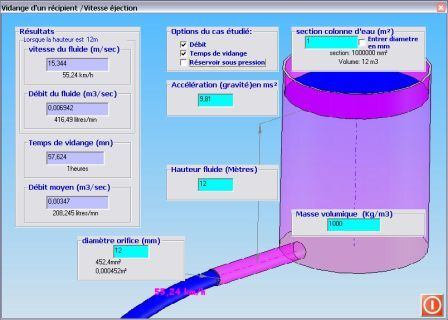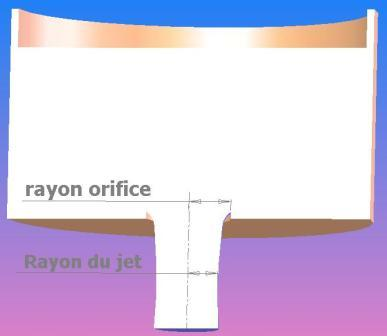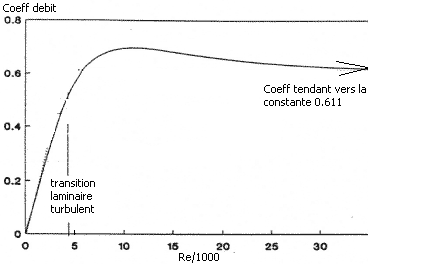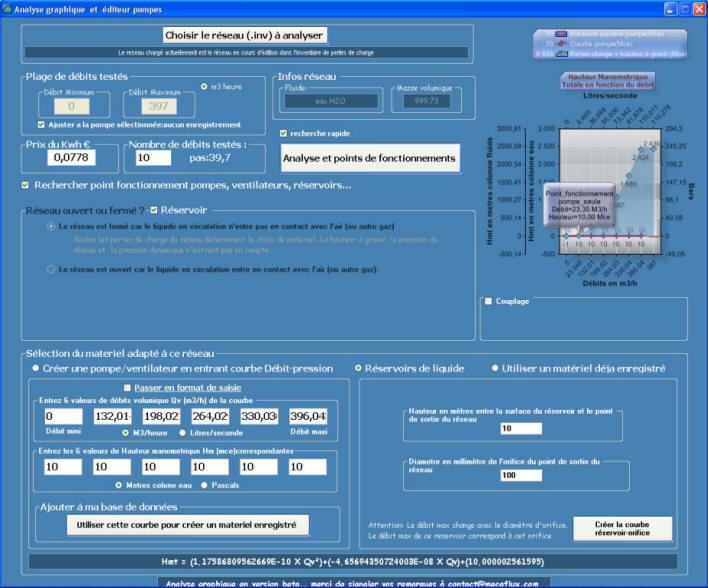Emptying a container is a classic theme of fluid mechanics.The calculation of the leakage flow of a retention tank of rainwater is an example often encountered, , Before turning to the formula for calculating ejection speed of a tank we will see in detail the example of a tank of water retention which the leakage rate is imposed (with mecaflux standard) :

In the case of a holding tank for rainwater, the tank must have an orifice which regulate the flow so as to not exceed the allowed leakage flow for the area.. This allowed leak rate is usually given by the municipal service or managers ditches outlets.To estimate the leakage rate allowed you can use two methods:

1. In the case of a direct release into the environment (ditch, river or otherwise) the leakage rate to take into account is 13l/s/ha built (maximum flow for the state XXXX).
Q (l/s) = 13( l/s/ha) x S(m²)
Q = leakage flow
S = Surface collected by the stormwater network
In this case, a written authorization of the gap outlet manager, or river (union, association ... etc..) Specifying that is able to absorb the additional flows generated by the project should be attached to the file
2. In the case of a direct release into the environment by infiltration, the leak rate to be considered, is the product of the permeability coefficient X basin area.
Q(m3/s) = K(m/s) x S(m²)
Q = leakage flow
K = permeability coefficient
S = surface of the basin
For the leakage rate in liters per second: Q (l/s) = Q(m3/s) x1000
In this case the permeability test must be attached to the file

Knowing the rate of leakage allowed you still have to calculate the diameter of the hole in the bottom of retention tank.This diameter determines the maximum flow rate that may leave the retention tank when completely filled.. We do calculate the maximum case, because if the tank is filled to half this rate will necessarily be lower.The tank volume does not affect the discharge rate, only the height of water in the reservoir and the diameter of the aperture determine the flow. The calculation interface emptying tank Mecaflux allows you to enter a filling height and diameter of the outlet tank. The discharge rate is calculated directly, then you only need to check if the discharge rate is less than the allowable leakage rate.

The volume of water collected, will have to pour in a tank with a capacity generating a height of maximum water causing a discharge flow rate, less than the leakage rate permitted. If the height of collected rainwater in your tank or retention tank generates an excessive flow rate, you have 2 solutions:

1. reduce the aperture (but this can cause an overflow if the volume of the tank is too small)
2. or reduce the load height (filling) of the container by increasing its surface area and / or volume.

In fact it is the ejection speed of the tank determines the flow.. Calculating the fluid velocity is a function of the height of water if the tank is in the open air.

Torricelli's formula gives us v = √2g.z

Where:

g is gravity(9.81ms²)

z is the height of water(m)

The emptying time is determined by the ratio between the sections of the tank and the orifice, and the flow rate varies over time depending on the depth of water remaining in the tank.

The formula for the integral gives : Emptying time= S/s.√2h/g

Where

g is the gravity(9.81ms²)

h is the height of water(m)

S the reservoir section

s section of the orifice

The output of the fluid being not over the entire surface of the orifice,(section of the jet is less than the section of the orifice)

the actual rate is lower than the theoretical throughput.

A flow coefficient (coefficient of discharge) is applied that checks: actual volume flow rate / theoretical throughput = coeff.in Mecaflux standard

Emptying time are calculated based on the output speed of the fluid, and the water height, variable over time, depending on the flow.

The ejection speed of the fluid from a reservoir under pressure, is calculated by selecting "pressurized tank" and entering the pressure

For the output rate (operating point) of a network, fed by a reservoir, taking into account head loss of the network, You need to know the operating curve of the tank and the operating curve of the network.
The intersection of the two curves gives the operating point..

curve of the network:

1. Calculate the pressure drop at an approximate rate (unimportant because the flow will be reviewed) elements of the segment connected to your tank with the inventory of losses.
click create curve tank: